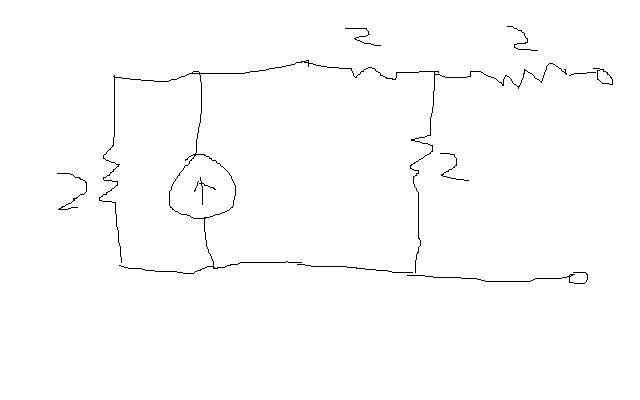# Thevenin equivalentFinding the thevenin equivalent of the above circuit

This is what I did, I set the current source to zero, no current passes through
so the Rth is the resistor on farthest right // with another 2 in series with 4.
so it is 5ohms?

Finding the open circuit voltage, I use a current divider(the current source is 10A given):
10 times ( 1/(2//2+2)) ) / (1/2 + 1/(2//2+2)) ) does it look alright to you guys? others solutions are appreciated

## Answers and RepliesFinding the thevenin equivalent of the above circuit

This is what I did, I set the current source to zero, no current passes through
so the Rth is the resistor on farthest right // with another 2 in series with 4.
so it is 5ohms?

Finding the open circuit voltage, I use a current divider(the current source is 10A given):
10 times ( 1/(2//2+2)) ) / (1/2 + 1/(2//2+2)) ) does it look alright to you guys? others solutions are appreciated

Your analysis is wrong. With the source open circuited, you have the rightmost resistor in series with the parallel of 2 and 4 ohm.
For the open circuit voltage, the source divides by a 2 ohm resistor on the left and 2+2 = 4 ohm in the right. The voltage is the current in the right arm times 2 ohm.

Your analysis is wrong. With the source open circuited, you have the rightmost resistor in series with the parallel of 2 and 4 ohm.
For the open circuit voltage, the source divides by a 2 ohm resistor on the left and 2+2 = 4 ohm in the right. The voltage is the current in the right arm times 2 ohm.

o so the Rth is 4//2 +2= 3.33 and since there is no current in the far right resistor, the current divider just involves the other 3 ?

hello johndoe,
yes the current divides between the three resistor and does not involve the rightmost resistor as no current flows because of open circuit.
And your Rth looks correct to me